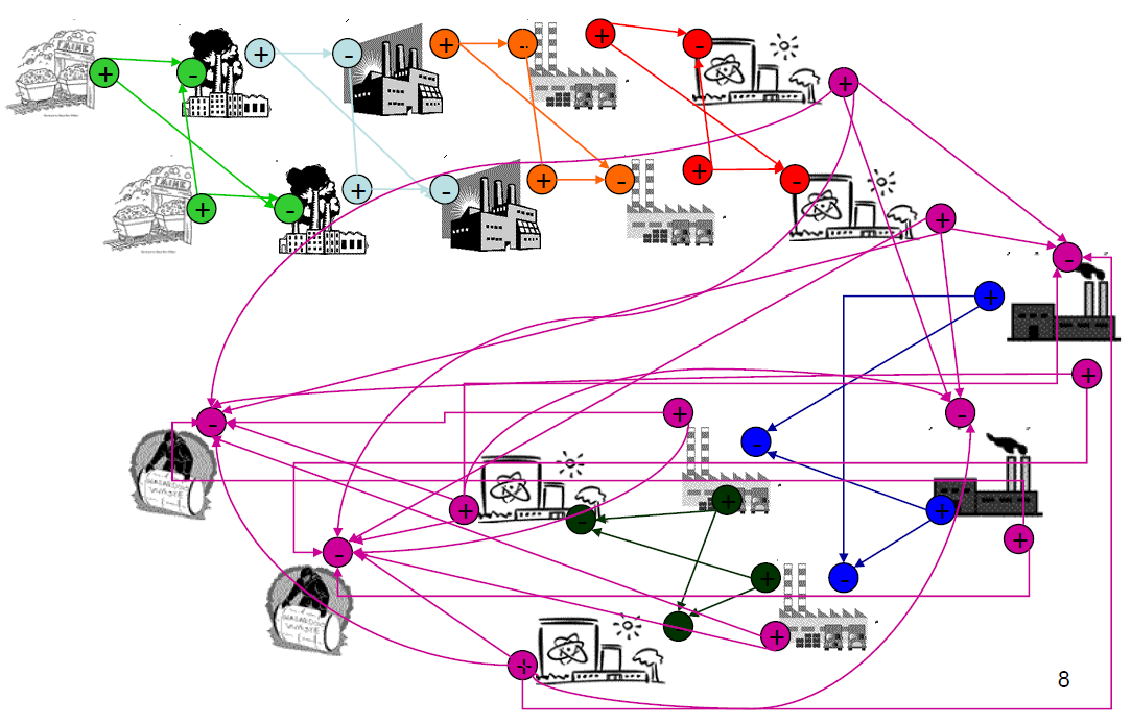# Data in Nuclear Engineering

### Kathryn (Katy) Huff$\phi(E,\vec{r},\hat{\Omega},T)$

$\phi(E, x, y, z, \theta, \omega, T)$$\sigma(x, i, E, T)$$\gamma(f, i)$$i$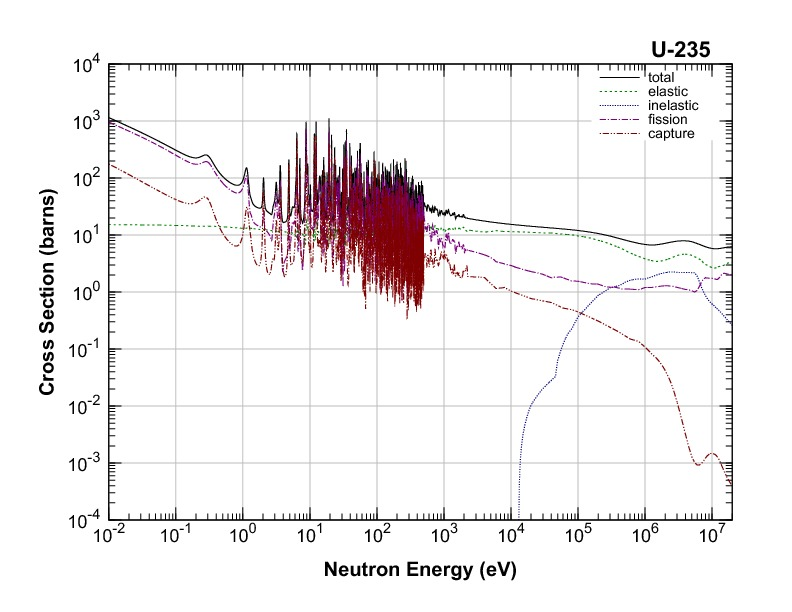$E$nuclear structure

## Evaluated Nuclear Data Sets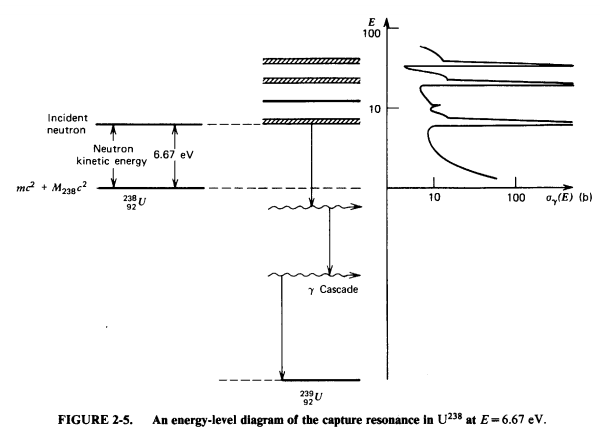$\sigma(x, i, E, T)=$ nuclear structure + experiments

## Experiments## Experiments## Nuclear Data is for Simulations## Simulation Methods

• Monte Carlo Methods
• Deterministic Methods
• Hybrid Methods
• Other keywords...
• lattice codes
• ray tracing algorithms
• acceleration schemes
• ...

## Application Specific Data Processing

• Energy discretization
• multigroup
• pointwise
• piecewise linear continuous
• Resonance integration
• ...

## How Do We Know It's Right?## The Data Dilemma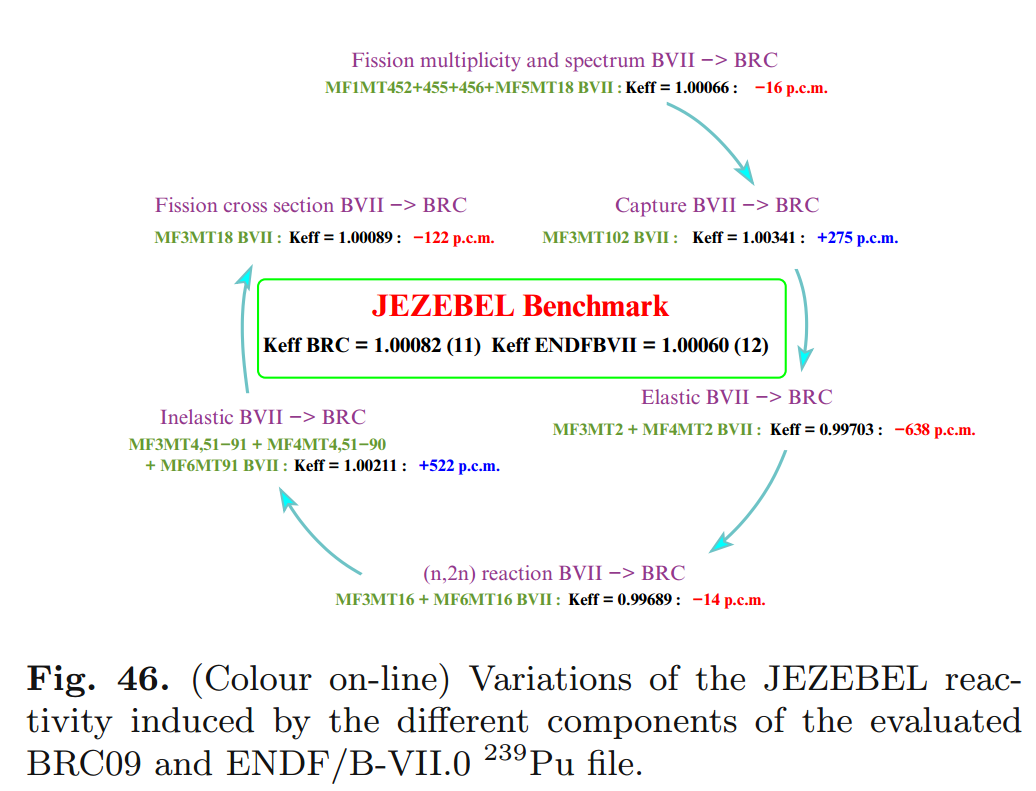## Unique Issues

Export control is serious.

# THE END

### Katy Huff

katyhuff.github.io/2016-10-13-info500Based on a work at http://katyhuff.github.io/2016-10-13-info500.

## A Few of My Favorite Things

• C++, Python, Fortran
• xml, markdown, rst, $\LaTeX$
• Doxygen, sphinx
• CMake, conda, macports
• hdf5, sqlite
• cython, boost, Coin
• jekyll, reveal.js, beamer
• yt, matplotlib, paraview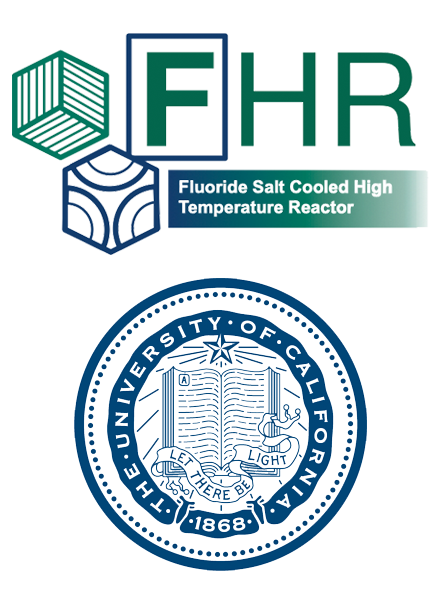## Transient Coupled Physics## Agent Based Systems Analysis

Hundreds of discrete facilities mine, mill, convert, fabricate, transmute, recycle, and store nuclear material.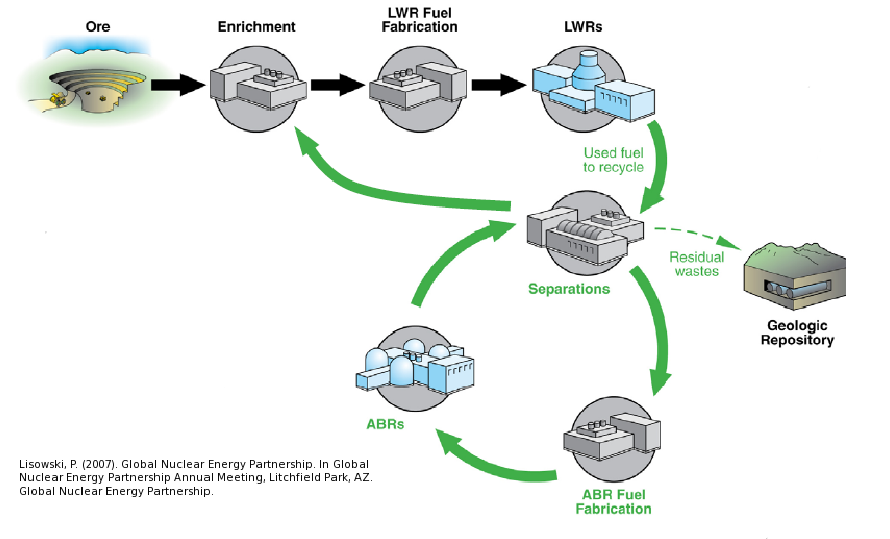## Agent Based Systems Analysis

A facility might create material.## Agent Based Systems Analysis

It might request material.## Agent Based Systems Analysis

It might do both.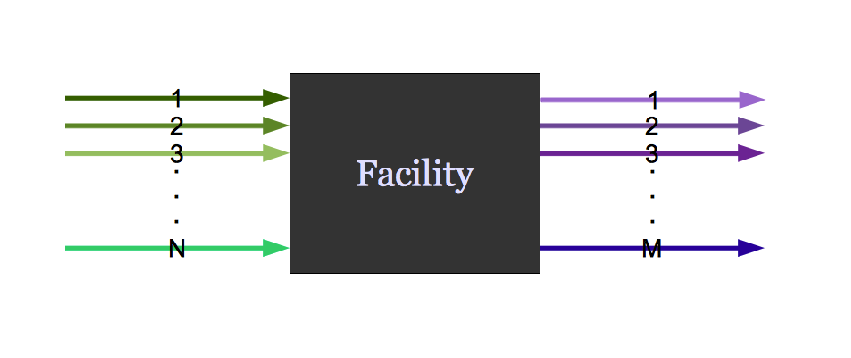## Agent Based Systems Analysis

Even simple fuel cycles have many independent agents.